Courses

# Retaining Wall and Earth Pressure Theories Civil Engineering (CE) Notes | EduRev

## SSC : Retaining Wall and Earth Pressure Theories Civil Engineering (CE) Notes | EduRev

The document Retaining Wall and Earth Pressure Theories Civil Engineering (CE) Notes | EduRev is a part of the SSC Course Foundation Engineering.
All you need of SSC at this link: SSC

Retaining Wall/earth Pressure Theories

CONTRASTING POINTS OF ACTIVE AND PASSIVE PRESSURES

 ACTIVE PASSIVE 1. Very little movement isrequired to mobilise theactive pressure.(about0.5% horizontal strain) 1. Much higher movementis required to mobilisethe pressure (about 2%of horizontal strain) 2. Failure plane is inclinedat 45º + Φ/2 2. Failure plane is inclinedat 45º – Φ/2 3. Width of sliding wedgeat the top of wall is H.Cot (45 + Φ/2) 3. Width of sliding wedgeat the top of wall is H.Cot (45 - Φ/2)

EARTH PRESSURE AT REST:
Earth pressure at rest rest is the horizontal pressure acting on the rigid structure.
Earth pressure is given by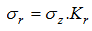For cohesive soils the Terzaghi formula for computing Kr is implemented in the program: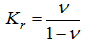where,
ν=poisson's ratio
For cohesionless soils the Jáky expression is used: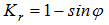where, Φ=Angle of internal friction of soil

For overconsolidated soils the expression proposed by Schmertmann to compute the coefficient of earth pressure at rest Kr is used: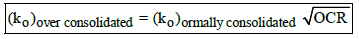where, OCR = Over Consolidation Ratio.
Typical values of Ko:
Type of soil - K o
Dense sand - 0.4 to 0.45
Loose sand - 0.45 to 0.5
Mechanically compacted sand - 0.8 to 1.5
Normally consolidated clay - 0.5 to 0.6
Over consolidated clay - 1.0 to 4.0

ACTIVE EARTH PRESSURE: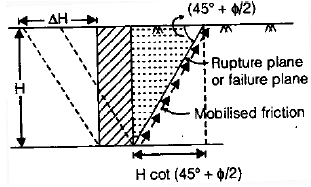ΔH = 0.2% to 0.5% of H
-length of failure block = H.cot(450+Φ/2)
-ΔH= 0.2%of H for dense sand.
- ΔH= 0.5%of H for loss sand.
-ΔH= 0.4% of H clay
Ka=(1-sinΦ)/(1+sinΦ)=tan2(450-Φ/2)
PASSIVE EARTH PRESSURE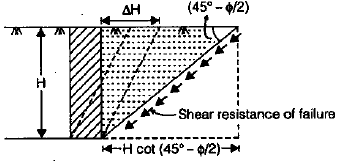Langth of failure block =H.cot(450-Φ/2)
- ΔH= 2% of H For dense sand
-ΔH=15% of H For losse sand
-ka . kp =1

where

kp = Coefficient of passive earth pressure.
Kp=(1+sinΦ)/(1-sinΦ)=tan2(450+Φ/2)
-Pa < Po < Pp

where, Pa = Active earth pressure.
Po = Earth pressure at rest.
Pp = Passive earth pressure.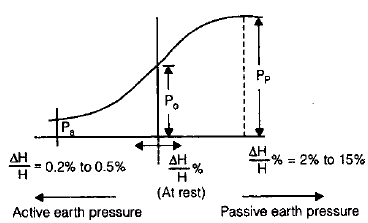ACTIVE EARTH PRESSURE BY RANKINE THEORY:

(i) Cohesionless soil on vertical smooth wall: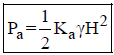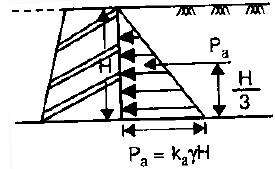acts at (H/3) from base.
where,Pa = Active earth pressure force on unit length of wall.

(ii) Submerged Cohesionless soil on vertical smooth wall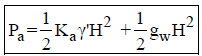acts at (H/3) from base.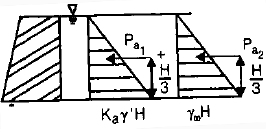where, g ' = Submerged unit weight of soil.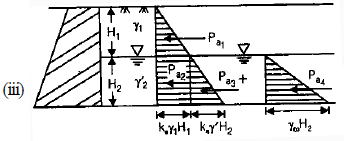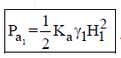acts at a  H2+H1/3 from base =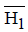Pa2 =Kaγ1H22acts at H2/2 from base =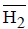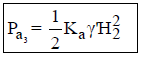acts at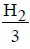from base =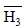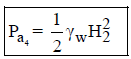acts atfrom base =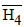Total Earth Pressure (Pa)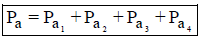acts at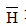from base
where,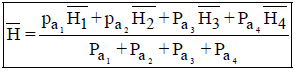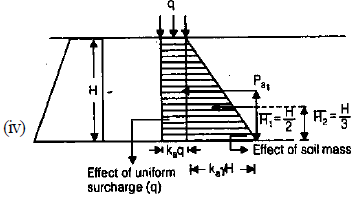Pa1 = kaq.H acts at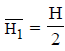from base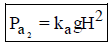acts at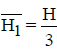from base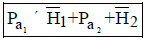acts at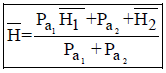from base.

(v)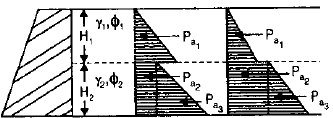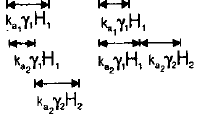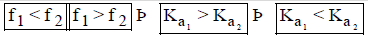•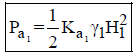acts at (H2 +H1) from base.
•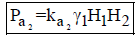acts at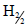from base.
•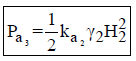acts at H2 from base.

(vi)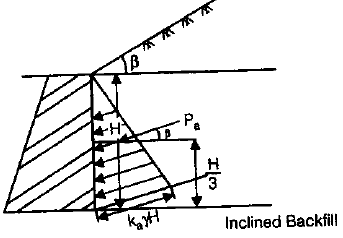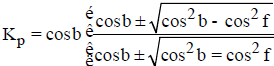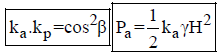acts at H/3 from base but line of ation is parallel is parallel to backfill.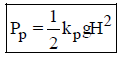acts at H/3 from base but line of action is parallel to backfill.
(vii)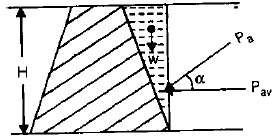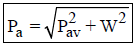where, W= Weight of soil over inclined back of wall of unit length.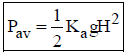Pav = Active earth pressure on imaginary wall of vertical back.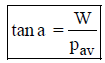pa = Resultant active earth pressure on the wall
a = Angle of inclination of resultant Pwith the horizontal.

(viii)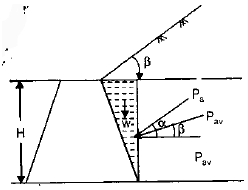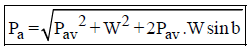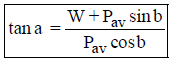where, Pav = Active earth pressure on Imaginary vertical back of wall. Acts parallel to back fill i.e., of b angle with hori zontal
W = Weigth of soil block of unit length.

ACTIVE EARTH PRESSURE FOR COHESIVE SOIL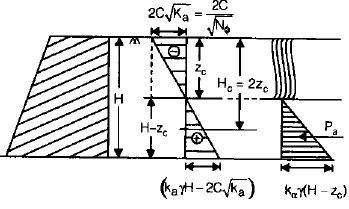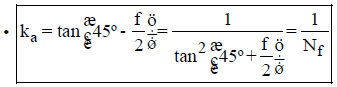whereNf = Influence Factor.

•   Active Earth Pressure of Any Depth z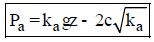• Active Earth Pressure of Surface. i.e., at z = 0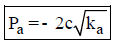• At Z = Zc ® Pa = O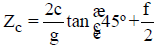where, ZC = depth of tension crack.

•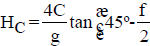where, Hc = Cirtical depth = 27º = max. Possible unsupported depth.

PASSIVE EARTH PRESSURE FOR COHESIVE SOIL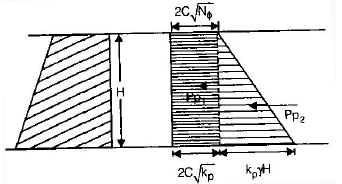• Passive Earth Pressure at any depth ‘Z’,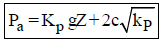• Total Pp on Unit Length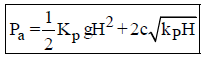• Coulombs Wedge Theory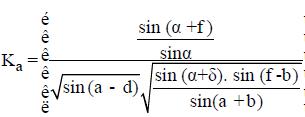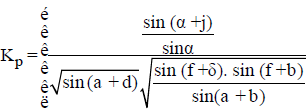where, a = Angle of back of wall with the hori zontal
b = Angle of sloping ground.
f = Angle of roughness between soil and wall
= 0º for smooth walls

• When Tension Cracks are not Developed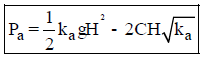acts at centroid of trapezoidal.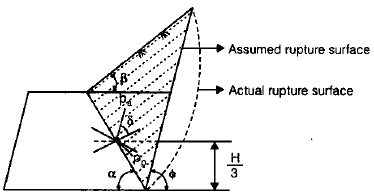• When Tension Cracks are Developed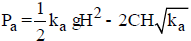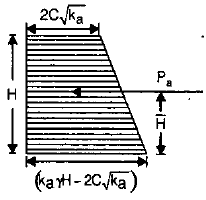Acts at centroid of trapezoidal

• When tension Cracks are Developed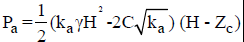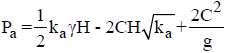or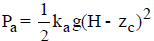acts at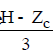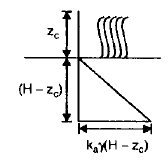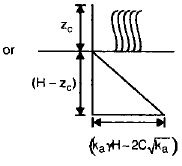Remember:- Cohesion decreases the active earth pressure while increases the passive earth pressure.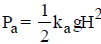acts at  (H/3) from base of an angledwith the normal of wall.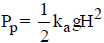Offer running on EduRev: Apply code STAYHOME200 to get INR 200 off on our premium plan EduRev Infinity!

## Foundation Engineering

18 videos|20 docs|7 tests

,

,

,

,

,

,

,

,

,

,

,

,

,

,

,

,

,

,

,

,

,

;